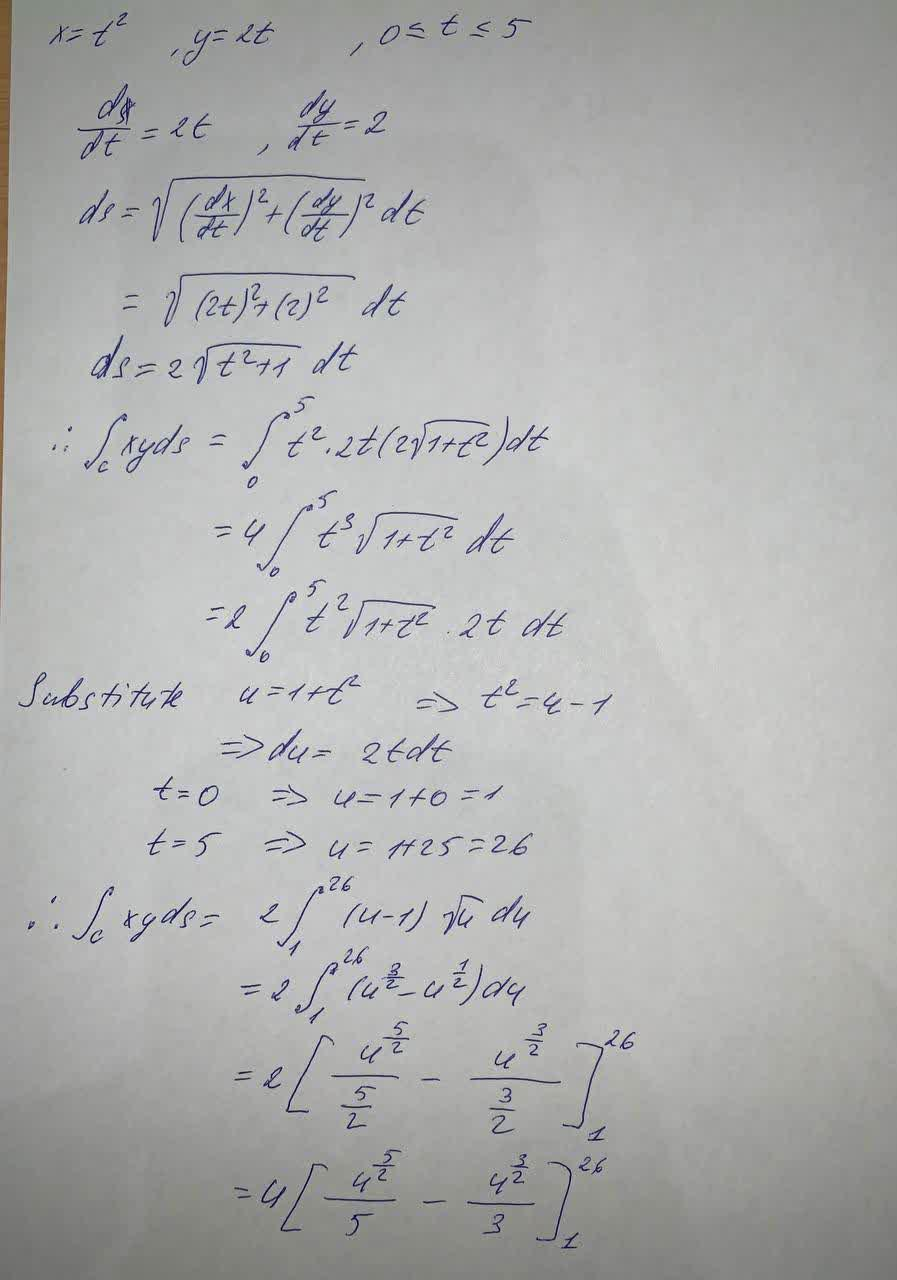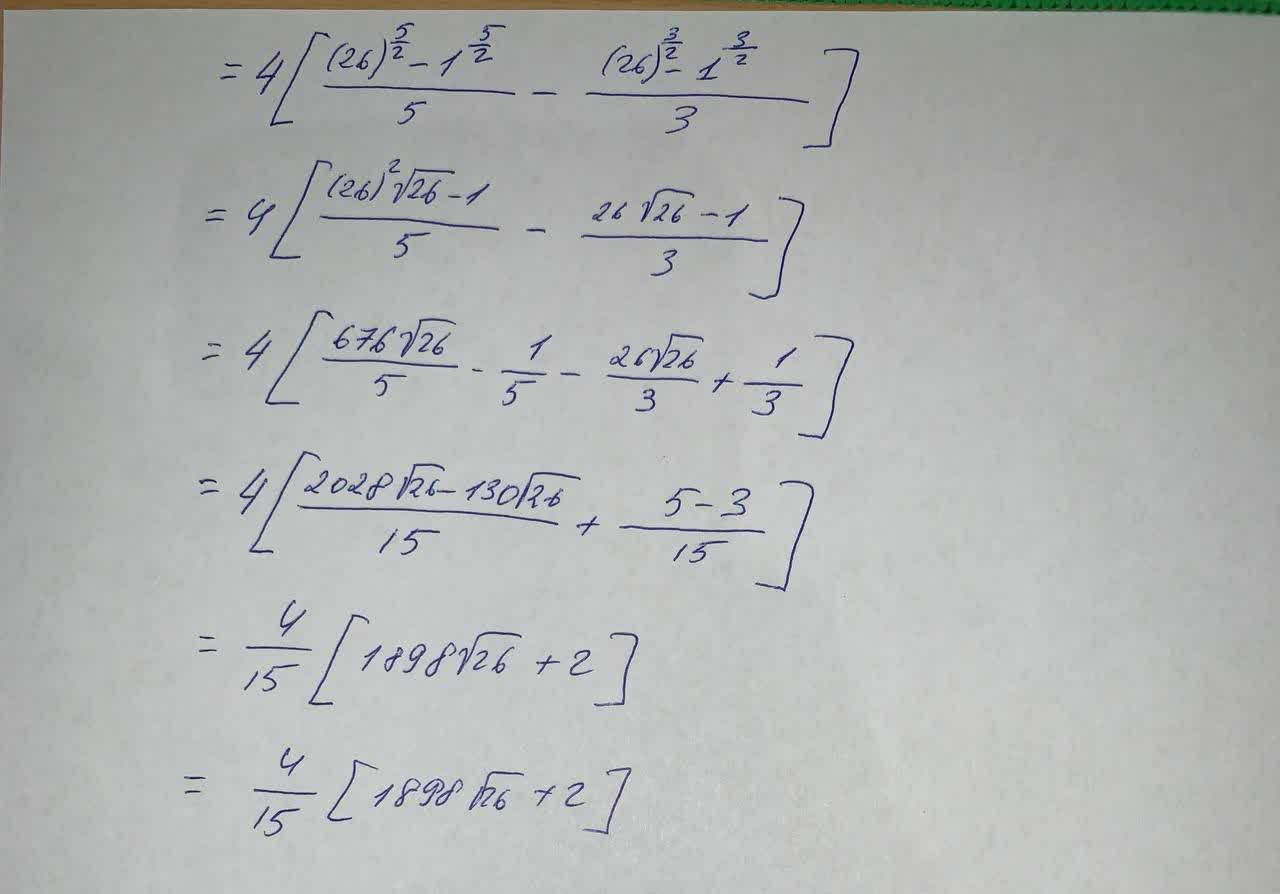# Evaluate the line integral, where C is the given curve C xy ds C: x=t^2 , y=2tAnonym 2021-09-16 Answered
Evaluate the line integral, where C is the given curve
C xy ds
C: $$\displaystyle{x}={t}^{{2}},{y}={2}{t},{0}\leq{t}\leq{5}$$

• Questions are typically answered in as fast as 30 minutes

### Plainmath recommends

• Get a detailed answer even on the hardest topics.
• Ask an expert for a step-by-step guidance to learn to do it yourself.d2saint0
To evaluate: